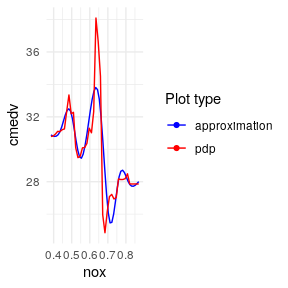Basic theory and usage

Motivation

In regression or classification problem, the main issue is choosing the model that should meet our requirements as much as possible. On the one hand, we want the chosen solution to have the best statistical properties such as accuracy, RMSE or R Squared - on the other hand, we focus on the interpretability of the model.

In the first case, the black box models, such as randomForest or XGBoost, perform better than others, while the second case is dominated by linear models. The xspliner package aims to combine both methods: use the knowledge gathered from black box models in order to build an interpretable linear one.

General idea

Below graphics show general idea used in xspliner model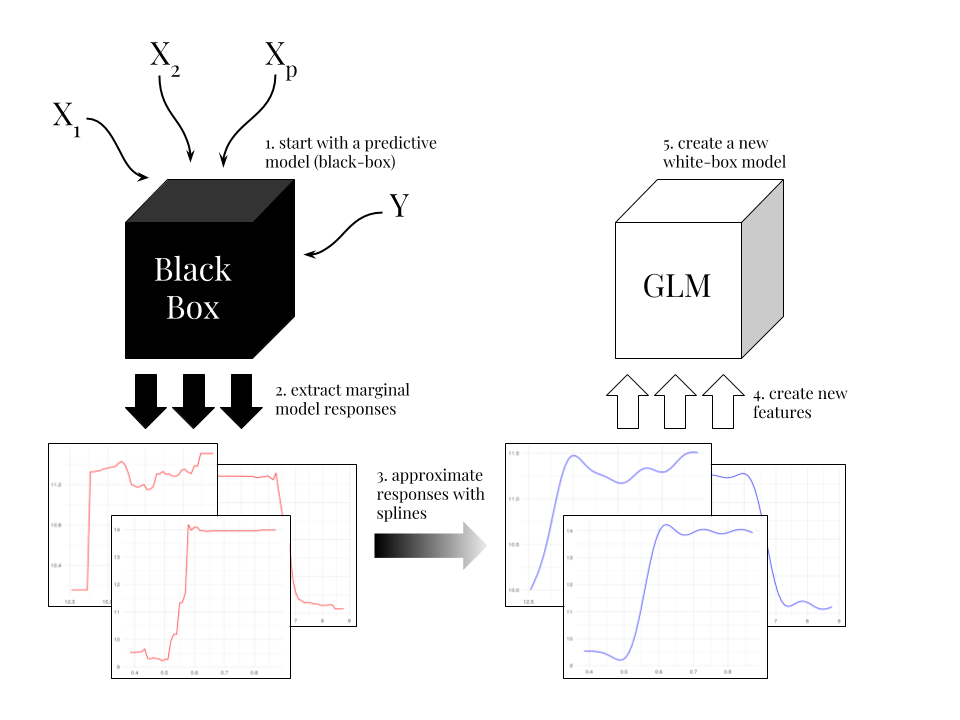Black box variable response

When black box model is already built, you may want to check how each predictor variable affects the final response. This is quite easy when you use a linear model or have low dimensional data (up to 2, 3 dimensions). One of the ideas for testing the predictor impact in more complicated model is to check an average model response of the selected variable.

One of such approaches called Partial Dependence Plots is implemented in the pdp package (Brandon M. Greenwell (2017). Pdp: The R Journal, 9 (1), 421-436.), or the ALEPlot package (Dan Apley (2017). ALEPlot: Accumulated Local Effects Plots and Partial Dependence Plots.) using Accumulated Local Effects Plots.

In each case, we get a single variable function, which should explain the impact of the predictor on the response variable.

The following pictures show the ptratio impact on cmedv in some random forest model based on the Boston Housing Data. Below curves are obtained by the approach of the pdp and ale methods respectively.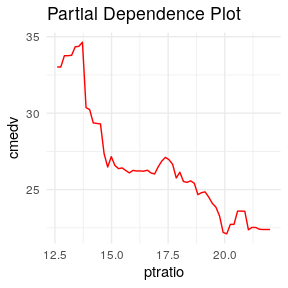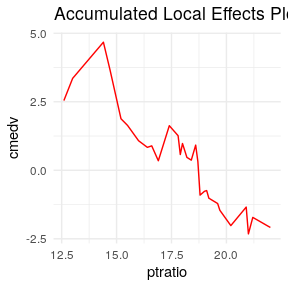As we can see, above functions are irregular, making it difficult to interpret explained effect.

If above functions had linear character, one would be tempted to approximate them with linear function. As a result it could be easy to interpret how it affects the variable explained in the black box model. What if the function is irregular, as above? Could we approximate it with polynomials?

Splines

Due to the large errors that occur with approximation of functions with polynomials, the approach using spline approximation is the most common solution. Splines (functions that are piecewise polynomials) have good approximating properties, in addition their form is overt so we can thus interpret the resulting function.

The following graphics show spline approximations of pdp and ale curves: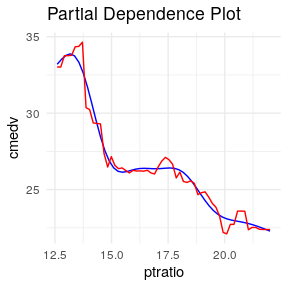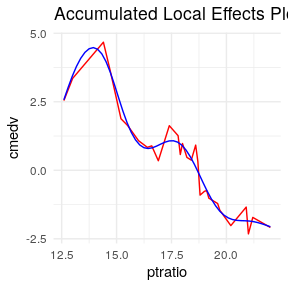But even with approximated PDP, how could we use it to interpret black box model?

Linear models based on response function and splines

The general idea of how to use the response function and splines to build an interpretable model that could be used as black box explainer is as follows.

• For each variable used in the black box model, create a response function based on one of the known methods, let’s mark it $$f_{x}$$
• Approximate $$f_{x}$$ using spline - the result is $$\widetilde{f}_{x}$$
• Build linear model, in which each predictor $$x_{i}$$ is transformed with approximated response function: $$\widetilde{f}_{x_{i}}(x_{i})$$

Shortly, using black box formula $y \sim x_{1} + \cdots + x_{n}$ use $y \sim \widetilde{f}_{x_{1}}(x_{1}) + \cdots + \widetilde{f}_{x_{n}}(x_{n})$ in linear one.

The resulting model uses a part of the information that was extracted while building black box model. If linear model performance is similar to black box one, it could be used as it’s good interpretation.

How to do it with xspliner?

Defining formula

This sections shows, how to build formula interpretable by xspliner package using Boston Housing Data from pdp package.

data(boston)
str(boston)
#> 'data.frame':    506 obs. of  16 variables:
#>  $lon : num -71 -71 -70.9 -70.9 -70.9 ... #>$ lat    : num  42.3 42.3 42.3 42.3 42.3 ...
#>  $cmedv : num 24 21.6 34.7 33.4 36.2 28.7 22.9 22.1 16.5 18.9 ... #>$ crim   : num  0.00632 0.02731 0.02729 0.03237 0.06905 ...
#>  $zn : num 18 0 0 0 0 0 12.5 12.5 12.5 12.5 ... #>$ indus  : num  2.31 7.07 7.07 2.18 2.18 2.18 7.87 7.87 7.87 7.87 ...
#>  $chas : Factor w/ 2 levels "0","1": 1 1 1 1 1 1 1 1 1 1 ... #>$ nox    : num  0.538 0.469 0.469 0.458 0.458 0.458 0.524 0.524 0.524 0.524 ...
#>  $rm : num 6.58 6.42 7.18 7 7.15 ... #>$ age    : num  65.2 78.9 61.1 45.8 54.2 58.7 66.6 96.1 100 85.9 ...
#>  $dis : num 4.09 4.97 4.97 6.06 6.06 ... #>$ rad    : int  1 2 2 3 3 3 5 5 5 5 ...
#>  $tax : int 296 242 242 222 222 222 311 311 311 311 ... #>$ ptratio: num  15.3 17.8 17.8 18.7 18.7 18.7 15.2 15.2 15.2 15.2 ...
#>  $b : num 397 397 393 395 397 ... #>$ lstat  : num  4.98 9.14 4.03 2.94 5.33 ...

We’re going to build model based on formula

cmedv ~ rm + lstat + nox

So let’s build a random forest black box model, that we use as a base for further steps:

boston_rf <- randomForest(cmedv ~ rm + lstat + nox, data = boston)

Now let’s specify which variables should be transformed. In this example only nox variable will use random forest effect (PDP or ALEPlot).

To indicate transformation use xs(nos) symbol.

So we have:

cmedv ~ rm + lstat + xs(nox)

This formula is enough to build a GLM model (with using default parameters and xspliner glm generating function). Nevertheless to understand deeply the approach let’s go further with the theory.

As the algorithm goes through creating black box based response function and its approximation we need to specify desirable parameters.

Specifying which method should we use to build response function

Let’s name one dimensional model response (such PD or ALE) as effect.

As remarked in first section, currently implemented effects for quantitative predictors are Partial Dependence Plots (pdp package) and Accumulated Local Effects Plots (ALEPlot package).

In order to configure one of this methods, we need to specify effect parameter inside xs symbol used in formula:

effect = list(
type = <method_type> # "pdp" or "ale",
... # named list - other parameters passed for chosen method
)

So to use PDP effect for the predictor just specify xs(nox, effect = list(type = "pdp")), for ALE xs(nox, effect = list(type = "ale")).

How to find out what other parameters can be used? Just check:

• pdp::partial in case of type = "pdp"
• ALEPlot::ALEPlot in case of type = "ale" the functions responsible for effect response.

Below we will use PDP random forest effect, that returns 40 response points for nox variable. By checking ?pdp::partial we can see that grid.resolution parameter specifies predictor grid.

Now we should just specify: xs(nox, effect = list(type = "pdp", grid.resolution = 40)).

Let’s verify correctness of this parameters with the bare usage of pdp::partial:

rf_effect <- pdp::partial(boston_rf, "nox", grid.resolution = 40)
#>         nox     yhat
#> 1 0.3850000 23.38131
#> 2 0.3974615 23.40352
#> 3 0.4099231 23.49511
#> 4 0.4223846 23.62153
#> 5 0.4348462 23.60978
#> 6 0.4473077 23.69874
nrow(rf_effect)
#>  40

We got data.frame with predictor and response values containing 40 rows. So parameter should correctly specify our expectations.

Remark

Here we can see that response function is presented as data.frame with two columns:

• nox - $$n$$ evenly spaced points across range of nox variable. Specified by grid.resolution parameter (51 by default)
• yhat - response function values on points specified in the first column

Let’s learn now how to specify approximation approach.

Specifying spline approximation parameters

Response function is approximated with mgcv::gam package and mgcv::s smoothing function.

xspliner allows using all smoothing methods provided by mgcv::s.

How can we do that?

Let’s name approximation result as transition. To specify it’s parameters such as spline base we can use transition parameter inside xs symbol. Similarly to effect, transition is specified as the parameters list. Possible options can be found by ??mgcv::s (a few extra options can be found in next articles).

Shortly:

transition = <mgcv::s parameters> # named list

Let’s assume we want to approximate response function with cubic splines and basis dimension equal to 10. As we can see in mgcv::s documentation, we need to set: k = 10 and bs = "cr".

We just need to use:

cmedv ~ rm + lstat + xs(nox, transition = list(k = 10, bs = "cr"))

Finally using both, we get the formula:

cmedv ~ rm + lstat + xs(nox,
effect = list(type = "pdp", grid.resolution = 40),
transition = list(k = 10, bs = "cr"))

To sum up, we specified formula for building GLM model, in which we transform nox variable with some function constructed on the following steps:

• we extract impact of nox variable on cmedv in already build black box model (actually not specified yet in formula)
• we get that impact as PDP in form of 40 evenly spaced (over nox range) points
• we estimate PDP with the use of cubic splines with 10 dimensional base The estimation is our final function used for nox transformation in final GLM.

Building the model

Having the formula defined, we almost have all the required data provided to build GLM. The only one left, that the approach requires is the black box model that is the basis of our resulting model.

We will use here model_rf random forest model that was built before.

The final step is to use xspliner::xspline to build the desired model.

xp_model <- xspline(
cmedv ~ rm + lstat +
xs(nox,
effect = list(type = "pdp", grid.resolution = 40),
transition = list(k = 10, bs = "cr")),
model = boston_rf
)

Lets check the model summary:

summary(xp_model)
#>
#> Call:
#> stats::glm(formula = cmedv ~ rm + lstat + xs(nox), family = family,
#>     data = data)
#>
#> Deviance Residuals:
#>      Min        1Q    Median        3Q       Max
#> -13.4873   -3.2935   -0.8747    1.9991   26.9926
#>
#> Coefficients:
#>              Estimate Std. Error t value Pr(>|t|)
#> (Intercept) -34.29942    5.05680  -6.783 3.32e-11 ***
#> rm            5.23681    0.41600  12.589  < 2e-16 ***
#> lstat        -0.52504    0.04355 -12.057  < 2e-16 ***
#> xs(nox)       1.31670    0.16255   8.100 4.20e-15 ***
#> ---
#> Signif. codes:  0 '***' 0.001 '**' 0.01 '*' 0.05 '.' 0.1 ' ' 1
#>
#> (Dispersion parameter for gaussian family taken to be 26.81888)
#>
#>     Null deviance: 42578  on 505  degrees of freedom
#> Residual deviance: 13463  on 502  degrees of freedom
#> AIC: 3106.2
#>
#> Number of Fisher Scoring iterations: 2

As the final model is just the GLM, the summary is also standard. One difference that we can see here is the formula call. It has missing xs parameters, and xs is treated as standard R function (previously it was just the symbol used in formula).

More details about can be found in following sections:

• Discrete response or predictor - learn how model works when black box is classifier or what effect and transition is used when predictor is discrete variable
• Graphics - learn how to plot the results, or compare compare xspliner with base black box.
• Automation - learn how to automate building xspliners without specifying your own formula and/or using global parameters
• Methods - learn about other xspliner methods and its environment
• Extras - what additional features are provided by xspliner
• Use cases - see xspliner in action with examples

Here we shortly show how the plot random forest effect and transition for nox variable:

plot_variable_transition(xp_model, "nox")